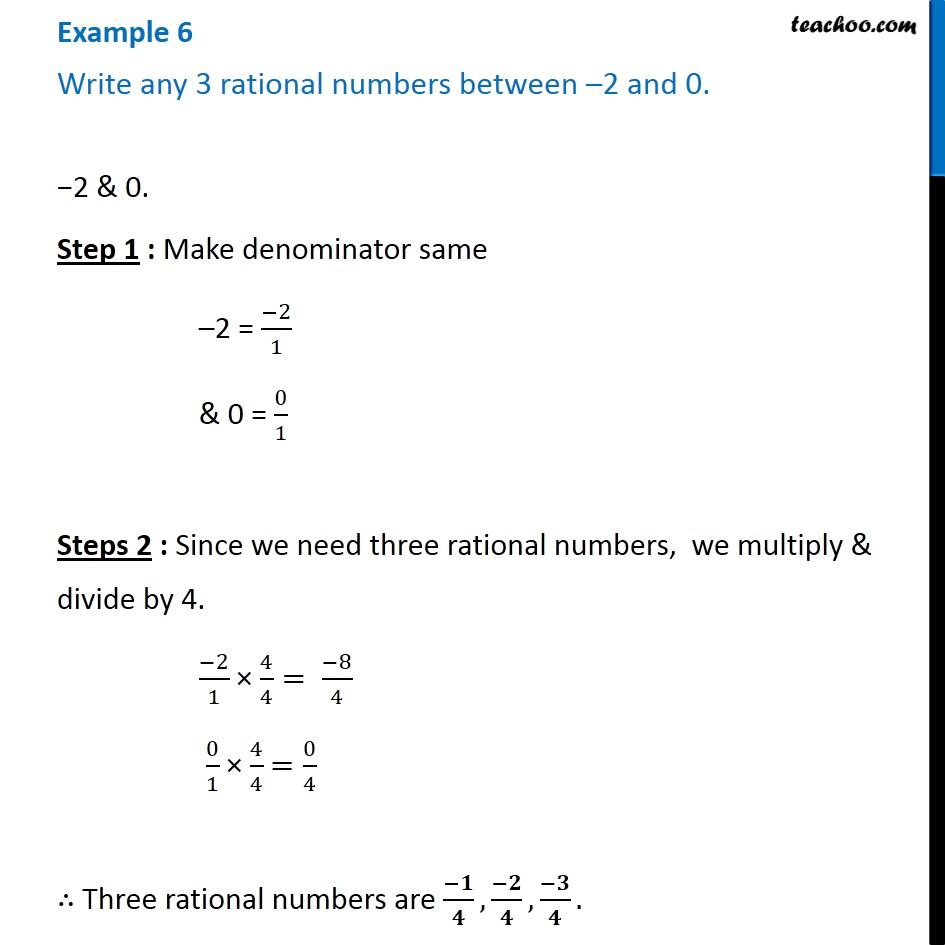Examples

Chapter 1 Class 8 Rational Numbers
Serial order wiseLearn in your speed, with individual attention - Teachoo Maths 1-on-1 Class

### Transcript

Question 3 Write any 3 rational numbers between –2 and 0. −2 & 0. Step 1 : Make denominator same –2 = (−2)/1 & 0 = 0/1 Steps 2 : Since we need three rational numbers, we multiply & divide by 4. (−2)/1 × 4/4= (−8)/4 0/1 × 4/4 =0/4 ∴ Three rational numbers are (−𝟏)/𝟒,(−𝟐)/𝟒,(−𝟑)/𝟒.